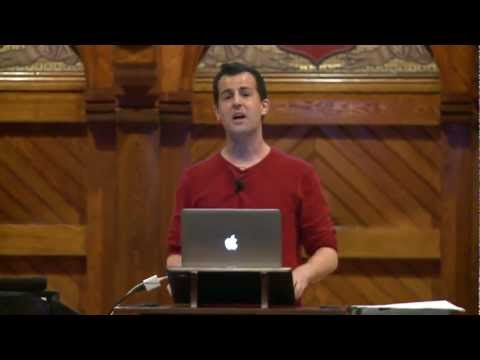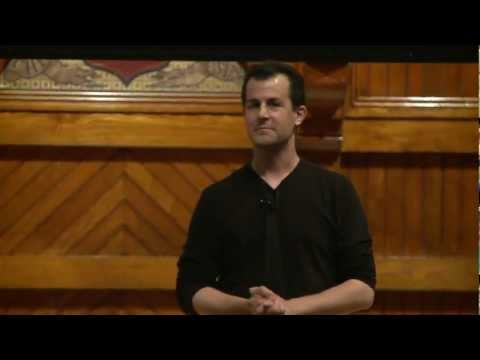# Conditions

## Notes

The conditionals and boolean expression blocks you've used in Scratch can all be recreated in C!

Conditions are important as they allow us to introduce logic into our programs.

Note how the code within this if block will only execute if the condition n > 0 evaluates to true. Conditions of if blocks are always boolean expressions that evaluate to either true or false. We’ll dive into boolean conditions in more detail on the next slide!

Boolean expressions like the ones on the left can only evaluate to boolean values of true or false. You can use them to compare values, e.g. 4 < 3 evaluates to false and 4 <= 3 evaluates to true.

Note that == is used to compare two numbers for equality.

! means "not", and negates the values of a boolean expression. So !(4 < 3) evaluates to true.

Additionally, in C, anything with a value of zero (e.g. int i = 0) evaluates to false, and any other value evaluates to true.

You can combine boolean expressions using the logical AND (&&) and OR (||) operators.

Here, invalid will print if the value of x is less than 0 or greater than 100, and valid will print if the value of x is between 0 and 100, inclusive.

We’ve seen that an if block can stand alone. It can also be paired with else.

If n > 0 does not evaluate to be true, the code within else will execute.

The important thing to remember is that the code within the if and else blocks are exclusive of each other: the else block will never execute if the if block already did! This may seem trivial for now, but you'll soon see how this logic can get a bit confusing.

But anyway, back to the code! 0 is not a negative number, so this program is flawed. We’ll fix it on the next slide.

The if... else construct can be extended to include three or more code blocks as in this slide's example.

Again, there's no way that more than one of those three printf statements can possibly execute, because once a condition is found to be true and its associated code executes, none of the other conditions will even be evaluated.

In contrast, multiple if blocks are not necessarily exclusive.

What will print if I enter the number 95?

Why?

How can we fix this bug?

This syntax is called a switch statement.

A switch statement takes a variable (n in this case) and determines which case statement will execute based on the value of that variable.

Finally, default defines behavior when no other case statement is executed.

** Note that switch statements can also be used on characters instead of integers. **

The ternary operator ?: is another kind of conditional.

Here's how it works:

• If n > 100 is true, the value to the left of the colon "high" is assigned to s.
• If n > 100 is false, the value to the right of the colon "low" is assigned to s.

So what will print out if the user inputs a value of 55?## Slides ( / )## Kids

Write a program that prompts the user to take a guess at the average number of children per family in the United States. Let the user know whether he/she got the answer right!

``````jharvard@run.cs50.net (~): ./a.out
Average number of children per family in US: 2
That is correct!``````
``````jharvard@run.cs50.net (~): ./a.out
Average number of children per family in US: 1
That is incorrect!``````

Try out some pseudocode here!
```#include <cs50.h>
#include <stdio.h>

int main(void)
{
// TODO
}
```

There’s a bug in this program such that if the user inputs the number 95, all four statements print out:

``````jharvard@run.cs50.net (~): ./a.out
You got an A!
You got a B!
You got a C!
You got a D!``````

Modify the program such that if the user inputs the number 95, only the correct statement will print out:

``````jharvard@run.cs50.net (~): ./a.out
You got an A!``````

Try out some pseudocode here!
```#include <cs50.h>
#include <stdio.h>

int main(void)
{
int n;

do
{
n = GetInt();
}
while (n > 100 || n < 60);

// TODO: fix the bug!
if (n >= 90)
printf("You got an A!\n");
if (n >= 80)
printf("You got a B!\n");
if (n >= 70)
printf("You got a C!\n");
if (n >= 60)
printf("You got a D!\n");
}
```

## Videos#### Monday, Week 1

From Scratch to CMore conditions